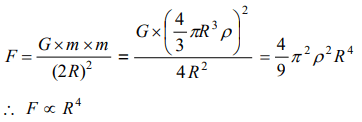## Gravitation Questions and Answers Part-3

1. Earth binds the atmosphere because of
a) Gravity
b) Oxygen between earth and atmosphere
c) Both (a) and (b)
d) None of these

Explanation: Gravity

2. Which of the following statements about the gravitational constant is true
a) It is a force
b) It has no unit
c) It has same value in all systems of units
d) It does not depend on the nature of the medium in which the bodies are kept

Explanation: It does not depend on the nature of the medium in which the bodies are kept

3. Two identical solid copper spheres of radius R placed in contact with each other. The gravitational attracton between them is proportional to
a) $R^{2}$
b) $R^{-2}$
c) $R^{4}$
d) $R^{-4}$

Explanation:4. Weightlessness experienced while orbiting the earth in space-ship, is the result of
a) Inertia
b) Acceleration
c) Zero gravity
d) Free fall towards earth

Explanation: Free fall towards earth

5. The time period of a simple pendulum on a freely moving artificial satellite is
a) Zero
b) 2 sec
c) 3 sec
d) Infinite

Explanation:6. Two planets have the same average density but their radii are $R_{1}$ and $R_{2}$ . If acceleration due to gravity on these planets be $g_{1}$ and $g_{2}$ respectively, then
a) $\frac{g_{1}}{g_{2}}=\frac{R_{1}}{R_{2}}$
b) $\frac{g_{1}}{g_{2}}=\frac{R_{2}}{R_{1}}$
c) $\frac{g_{1}}{g_{2}}=\frac{R_1^2}{R_2^2}$
d) $\frac{g_{1}}{g_{2}}=\frac{R_1^3}{R_2^3}$

Explanation:7. An iron ball and a wooden ball of the same radius are released from a height ‘h’ in vacuum. The time taken by both of them to reach the ground is
a) Unequal
b) Exactly equal
c) Roughly equal
d) Zero

Explanation:8. The correct answer to above question is based on
a) Acceleration due to gravity in vacuum is same irrespective of size and mass of the body
b) Acceleration due to gravity in vacuum depends on the mass of the body
c) There is no acceleration due to gravity in vacuum
d) In vacuum there is resistance offered to the motion of the body and this resistance depends on the mass of the body

Explanation: Acceleration due to gravity in vacuum is same irrespective of size and mass of the body

9. When a body is taken from the equator to the poles, its weight
a) Remains constant
b) Increases
c) Decreases
d) Increases at N-pole and decreases at S-pole